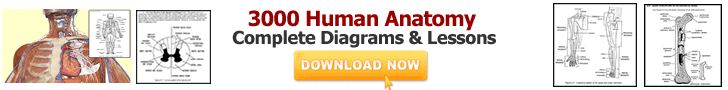# Numbers In Words## Numbers In Words Lessons and Worksheets for Grade 2

Writing numbers in words is a key number sense skill which is a step towards writing and recognizing numbers in different forms such as expanded, word, and standard form.

How to write numbers in words and number spellings is a must know skill for kids in second grade math. On this page students are going to learn how to write numbers in words form from standard form or vise versa.

To develop this key skill, students are shown examples of numbers written out in word form in the beginning of each of our worksheet. Then kids are asked to complete rest of the worksheet, independently.

There are ten basic worksheets to learn writing numbers in words and number spelling for grade two math students. Counting numbers or natural numbers can be written using many forms, but main forms are writing number in words, writing numbers in standard form (which is also known as writing numbers using numerals or digits) and finally writing numbers in expanded form.

 A key skill for 2nd grade math students is, to learn how to write the numbers in words when the standard form of the number is given. In grade two math; kids should know how to spell numbers up to twenty and few other numerals in words, such as thirty (30), forty (40) and fifty (50) and so on. Once kids have mastered the basic number spellings skill, the skill to write larger number spelling comes automatically by practice. Below are the key lessons and worksheets on writing numbers in words for 2nd grade math. Print them all and use them to teach the skill to your kids if you are a parent, or for your students if you are a teacher.

### Worksheets to practice writing numbers in words from standard form

 Hope that you have printed all of the above worksheets on writing numbers in words when the standard form is given. After learning to write numbers in words, the next step is to practice writing numbers in other forms. The other most important form of writing numbers is to write the numbers in standard form when somebody say the number. Or in other words, write a number by listening to a person. For example; when I say, fifty; a 2nd grade math student should be able to write the number 50, and same is true for three digit numbers or hundreds. More example are given below: Forty Five = 45;         Ninety six = 96;           Two hundreds and fity = 250 Two thousands six hundreds = 2,600;          Nine thousands ninety = 9,090

 Our next stop is to practice worksheets on writing numbers in standard form, when the numbers in words are given or somebody tell you to write a number. Below are the key worksheets on writing numbers in standard form, when numbers in words are given: Print the above worksheets on numbers in words and practice these again and again to obtain excellent marks in 2nd grade math and beyond. We wish you all the best in your math learning, great success in your studies and life forever.

 @import url(http://www.google.com/cse/api/branding.css);Custom Search